Select Page

Difficulty Level = 5 [What’s this?]

UPDATE: I have re-done this project using simple 434MHz RF transmitter/receiver devices. Check out the new project!.

UPDATE: Also see this project for an easy way to display a temperature reading: Digit Shield Temperature Display.

I decided to explore the more advanced features of XBee radios by building a remote temperature sensor. You can get quite a bit of control over an XBee radio without a microcontroller at all. You can configure the radio to send sensor readings at particular intervals when it detects changes on certain input pins. For the details on configuring XBee radios, see the documentation at Digi International.

Input pin 19 on my sensor radio is configured (parameter D1) with value ‘2’ which means that it will read analog input, and the IO sampling rate (parameter IR) is set to ‘1000’ which sends a sample every 4096ms.

An LM34 temperature sensor outputs a variable voltage depending on the temperature. The mapping is extremely simple: 10mV for every Fahrenheit degree. So, at 72 degrees F, the output is 720mV.

Why did I choose pin 19? I started with pin 20, but I burned it out. The pins can only handle an analog input of up to 1.2V, and I think I may have sent too much into the pin. How? Well, let’s say it involved holding a cold Pepsi can on the circuit to cool off the temperature sensor, and I shorted out a connection with the can. Oops. I’m lucky I didn’t burn out the entire XBee chip.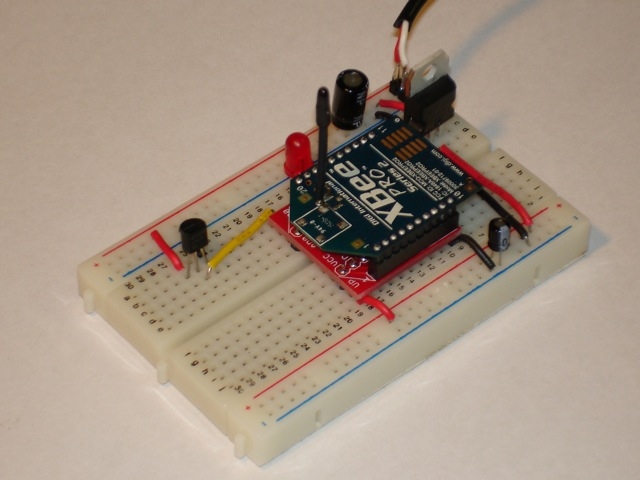Remote temperature sensor

Here is the circuit for the remote sensor: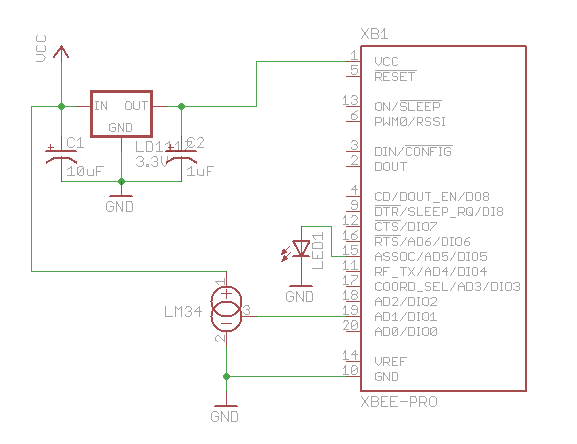Schematic for remote sensor circuit

For the receiving side, I used an Arduino with an XBee shield and a two digit LED display: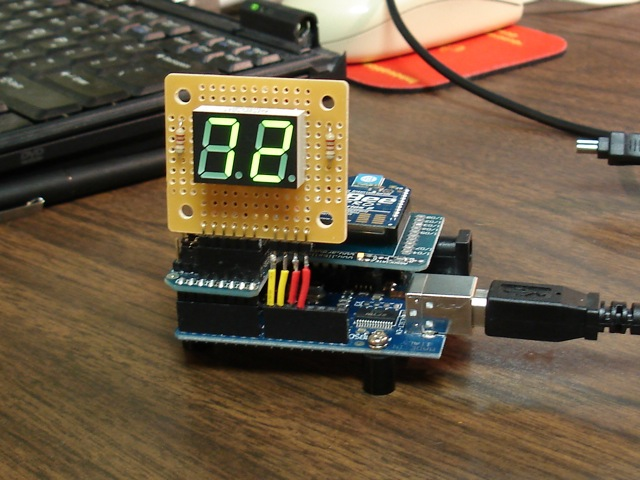Arduino with XBee shield and LED display

Here’s the Arduino code for reading incoming packets from the XBee and displaying the received analog sample on the LED display. Parsing an XBee packet is quite complex, unfortunately. But after studying the documentation for a while, it isn’t that hard.

#define NUM_DIGITAL_SAMPLES 12
#define NUM_ANALOG_SAMPLES 4

int groundPins = {8, 2, 3, 4, 5, 9, 7};
int digitPins = {11, 10};
int ON = HIGH;
int OFF = LOW;
int number;
int digit;
int TOP = 0;
int UPPER_L = 1;
int LOWER_L = 2;
int BOTTOM = 3;
int LOWER_R = 4;
int UPPER_R = 5;
int MIDDLE = 6;

int index;
int n = 0;

int packet;
int digitalSamples[NUM_DIGITAL_SAMPLES];
int analogSamples[NUM_ANALOG_SAMPLES];

void setup()
{
Serial.begin(9600);

for(int i=0;i<7;i++) {
pinMode(groundPins[i], OUTPUT);
}
for(int i=0;i<2;i++) {
pinMode(digitPins[i], OUTPUT);
}
initNumber();

setDigit(n);
}

void loop() {
drawDisplay();
}

if (Serial.available() > 0) {
if (b == 0x7E) {
packet = b;
int dataLength = (packet << 8) | packet;

for(int i=1;i<=dataLength;i++) {
}
int apiID = packet;

printPacket(dataLength+4);

if (apiID == 0x92) {
int analogSampleIndex = 19;
int digitalChannelMask = (packet << 8) | packet;
int d = (packet << 8) | packet;
for(int i=0;i < NUM_DIGITAL_SAMPLES;i++) {
digitalSamples[i] = ((d >> i) & 1);
}
analogSampleIndex = 21;
}

for(int i=0;i<4;i++) {
if ((analogChannelMask >> i) & 1) {
analogSamples[i] = (packet[analogSampleIndex] << 8) | packet[analogSampleIndex+1];
analogSampleIndex += 2;
} else {
analogSamples[i] = -1;
}
}
}
}

int reading = analogSamples;  // pin 19

// convert to Fahrenheit.  10mv per Fahrenheit degree
float f = v / 10.0;

// round to nearest int
n = (int)(f+0.5);
setDigit(n);
}
}

void drawDisplay() {
for(int g=0;g<7;g++) {
digitalWrite(groundPins[g], LOW);
for(int i=0;i<2;i++) {
if (digit[i] < 0) {
continue;
}
digitalWrite(digitPins[i], number[digit[i]][g]);
}
delay(0);  // for some reason, this is required even if the value is 0
digitalWrite(groundPins[g], HIGH);
}
}

void setDigit(int n) {
n = n % 100;
digit = n % 10;
digit = (n / 10) % 10;
if ((digit == 0) && (n < 10)) {
digit = -1;
}
}

void initNumber() {
number = ON;
number = ON;
number = ON;
number = ON;
number = ON;
number = ON;
number = OFF;

number = OFF;
number = OFF;
number = OFF;
number = OFF;
number = ON;
number = ON;
number = OFF;

number = ON;
number = OFF;
number = ON;
number = ON;
number = OFF;
number = ON;
number = ON;

number = ON;
number = OFF;
number = OFF;
number = ON;
number = ON;
number = ON;
number = ON;

number = OFF;
number = ON;
number = OFF;
number = OFF;
number = ON;
number = ON;
number = ON;

number = ON;
number = ON;
number = OFF;
number = ON;
number = ON;
number = OFF;
number = ON;

number = ON;
number = ON;
number = ON;
number = ON;
number = ON;
number = OFF;
number = ON;

number = ON;
number = OFF;
number = OFF;
number = OFF;
number = ON;
number = ON;
number = OFF;

number = ON;
number = ON;
number = ON;
number = ON;
number = ON;
number = ON;
number = ON;

number = ON;
number = ON;
number = OFF;
number = ON;
number = ON;
number = ON;
number = ON;
}
void printPacket(int l) {
for(int i=0;i < l;i++) {
if (packet[i] < 0xF) {
// print leading zero for single digit values
Serial.print(0);
}
Serial.print(packet[i], HEX);
Serial.print(" ");
}
Serial.println("");
}

while (true) {
if (Serial.available() > 0) {
}
}
}

Here’s how I wired the back of the LED display. The code displays each digit separately to save Arduino pins but toggles between the digits so fast you can’t see any flicker.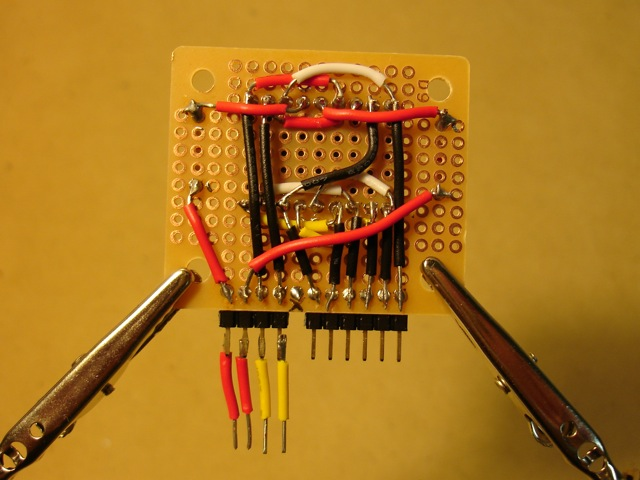Back of LED display. The pin positions are designed to fit the XBee shield.

Here’s how it looks in the dark!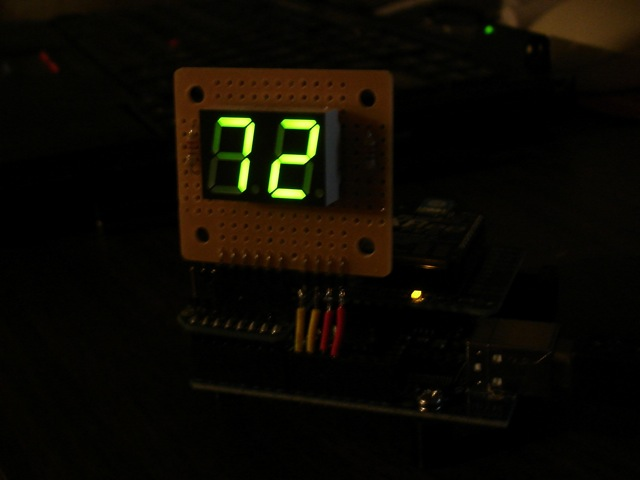In the dark!